American Journal of Modern Physics
Volume 3, Issue 6, November 2014, Pages: 247-253

Unified field theory and topology of atom

Zhiliang Cao1, 2, *, Henry Gu Cao3, Wenan Qiang4

1Wayne State University, College of Engineering W Warren Ave, Detroit, USA

2Shanghai Jiaotong University, School of Materials Science and EngineeringShanghai, China

3Northwestern University, Weinberg College of Arts and Sciences Clark St, Evanston

4Northwestern University, Robert H Lurie Medical Research Center Room, E Superior, Chicago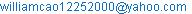(Zhiliang Cao)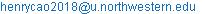(H. G. Cao)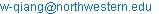(Wenan Qiang)

Zhiliang Cao, Henry Gu Cao, Wenan Qiang. Unified Field Theory and Topology of Atom. American Journal of Modern Physics. Vol. 3, No. 6, 2014, pp. 247-253. doi: 10.11648/j.ajmp.20140306.18

Abstract: The paper "Unified Field Theory and the Configuration of Particles" opened a new chapter of physics. One of the predictions of the paper is that a proton has an octahedron shape. As Physics progresses, it focuses more on invisible particles and the unreachable grand universe as visible matter is studied theoretically and experimentally. The shape of invisible proton has great impact on the topology of atom. Electron orbits, electron binding energy, Madelung Rules, and Zeeman splitting, are associated with proton’s octahedron shape and three nuclear structural axes. An element will be chemically stable if the outmost s and p clouds have eight electrons which make atom a symmetrical cubic.

Keywords: Unified Field Theory, Quantum Field Theory, Standard Model, Zeeman Effects, Madelung Rules

1. Introduction

Unified Field Theory (UFT) [1-8] predicts that a proton has an octahedron  shape. The positive electronic charge forces from nuclei are vertical to the faces of the octahedron. The electrons of an atom are interacting with the tri-axes of the protons.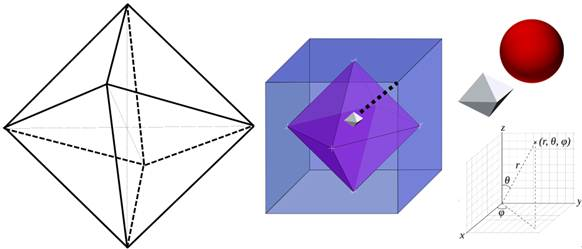Figure 1. Octahedron Proton and Electron Movements

Two electrons with mirrored moving direction and speed at the opposite side of the nucleus are paired electrons. The pairing increases the structural stabilities of nucleus. The ground state electrons in an atom will first meet pairing requirements before taking a new orbit.

Three axes of octahedron proton bounce back the electron’s mass wave and create triangle grid wave pattern. The electron "orbits" are nodes on the grids. Each node maps to a Zeeman splitting line. When electrons on nodes along the central line of the triangle wave pattern have positive spin, the Zeeman splitting lines go downward when strong magnetic field strength increases, otherwise, Zeeman splitting lines go upward.

Madelung rules can be explained by the propensity based on main orbit number plus azimuthal distortion times the interactive forces acting on axes of proton. The exceptions of the rule are results of unsymmetrical nuclear topological bases.

A simple experiment is designed to demonstrate the diamond grid interference pattern. A camcorder, and squared water bucket can be used to perform such experiment.

2. Results

2.1. Electron Movements

If the proton is in octahedron shape , the face of octahedron proton not only gives nuclei their topologies , but also carves the configuration of electrons. One of the issues associated with Schrödinger equation is that the equation does not consider the topology of the nuclei as nuclear structure is not "clear". In UFT [1-4], the waves are part of state that is stabilized by resonance. In atom, mass waves of electron under nuclear topology create stable wave pattern the electron "orbit" is based on. If the nuclei are in octahedron shape [1-2], the electronic mass waves’ primary direction is vertical to the eight faces of the octahedron. Logically, an electron collides with one of eight faces of proton and bounces like a ball. Even though the mass waves are possibility waves, an electron has a steady straight line "orbit" from nucleus to a fixed high possibility point of wave pattern.

When the atomic number is one, the nuclei of hydrogen isotopes are shaped as dot or line. The movement of electron makes the nucleus constantly spinning in the gas state in normal temperature. Helium isotopes have line shaped nucleus. When the atomic number (proton count) is greater than two, the nuclei become 2D symmetrical plate or 3D octahedron piles.

2.2. Squared Atom

The electrons are bouncing against a face of nuclear octahedron pile as their main "orbit".

When each octahedron face has an outer shell electron vertical to the face, the outer shell electrons form a cubic. Only noble gas elements with eight out most s/p clouds (except helium) form perfect cubic shape.

2.3. Axes Diffraction and Electron Orbits

The three axes of nucleus interact with the electron mass waves and create diffraction pattern that is similar to the crystalline diffraction. The charged axis has electronic field interaction as well, it double the wavelength of mass wave associated with charged axis. The proton/electron diffraction pattern shifts to charge oriented pattern, and topology center of the grid shifts to center of p cloud. Various electron clouds are related to the layers of triangle strips. Each triangle node represents an "orbit". Each node is mirrored with a node on the opposite side of proton face.

p: 3*2, d: 5*2, f: 7*2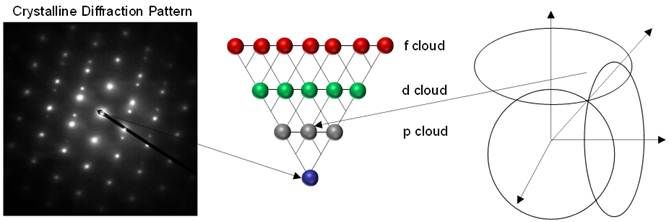Figure 2. Electron Diffraction Triangle Grids and Zeeman Splitting

Electrons are evenly distributed vertical to eight octahedron faces. The possibility of the various electron clouds are based on the strength of electron’s mass wave. The cloud s has highest priority for its high possibilities. The priority order is:

s, p, d and f

2.4. Spin

Based on the topology location of an "orbit" in triangle grid, an electron can be located topology balanced location or unbalanced ones. The electrons are interacting with weak waves. Smallest unit of weak wave (1/137.036)* (1/137.036) is ½ spin.

2.5. Zeeman Splitting

During Zeeman splitting [9-12], when element is in gas or liquid phase, a strong magnetic redshift up spin photonic electron photonic spectrum and blueshift down spin electron photonic spectrum due magnetic resonance of nucleus and electron.

2.6. Quantum Numbers

The main movement of the electrons in atom is vertical to one of eight faces and each electron collides with a proton as one proton can associate with multiple electrons. Each face of octahedron proton or neutron is a triangle hole with axes as boundaries. The charged field of electron crashes into the triangle hole, interacts with axes, and comes out with opposite moving direction.

Therefore, an electron’s movements can be studied using Spherical coordinate system FIG 1. Assume that Z axis is the charged axis, X and Y are uncharged ones.

When the electron is in the space, where x > 0, y>0 and z>0 and its movement is not perfectly vertical to the octahedron faces, q and j is direction and r is distance to nucleus:

The movement in three directions interacts with the nuclear octahedron axes, x, y and z. The quantum number along the r direction interacting with octahedron face is n, along q direction interacting with z is l and along j interacting with x and y is ml.

In the ground state, the n wave is the energy level, or main wave:

n=1,2,3,…

Along q, an electron positions on the grid line of n-1, n-2,…, 0. This is quantum number l.

Once the direction of n and l is decided, the interference high possibility nodes can be l, l-1, l-2, 0, -1, -2,…-l. This is quantum number ml.

2.7. Pauli Exclusion Principle

When proton is in octahedron shape, the electron mass waves are interfering with any face except the opposite face. Assume that A(x, x) represents movement along an octahedron face O, since movement is vertical to O:

A(x, x) = 0

A paired electrons on the opposite side of octahedron face are A(x, y) and A(y, x). The movements are opposite:

A(x, y) = -A(y, x)

It follows Pauli exclusion principle [13-17].

Even though Pauli exclusion principle is mainly for wavefunctions [18-30], the physical mass waves are used as above to discuss the principle.

When the same wave on two octahedron faces that are not opposite to once another, they are for the same electron interaction.

2.8. Energy Levels

The energy of an electron is:

0.510998928 * 106 eV

When an electron collides with the nucleus, it interacts with the weak waves [1-3] in the nucleus with energy of:

(0.510998928 * 106)/(137.036*137.036) =27.211 eV(38)

Electron loses half of energy above as electron’s charge energy is half of total energy. The rest of energy participates weak interaction:

27.211/2 = 13.605 eV(69)

One electron, one proton (atomic number=1), lowest energy:

E = -13.605 eV(69)

One electron, more proton (atomic number=Z), closest to nucleus. More proton increases weak wavelength [1-3] to factor of Z:

2E = - (0.510998928 * 106)/((137.036/Z)*(137.036/Z))eV

E = -Z2 13.605 eV(69)

When an electron interacts with Z protons in nucleus at n shell, the energy will be: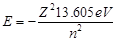2.9. Hydrogen’s Binding Energy Study

Hydrogen’s electron binding energy experimental value is 13.59844 eV.

A proton mass is 1836.15 times of electron mass. When an electron collides with proton, the momentum is 1/1836.15 of electron momentum relative to electron and the absolute momentum is 1/1837.15. When the electron momentum is 1, the total momentum is (1+1/1837.15). The binding energy is related to absolute momentum, and has correction factor of 1/(1+1/1837.15).

Proton has a weak resonance binding wave of (5/4)/(137*137*2*3) . The weak wave provides additional binding energy by factor of (5/4)/(137*137*2*3).

13.60569*(1+(5/4)/(137*137*2*3))/(1+1/1837.15) = 13.59844 eV

The above calculated value based on electron collision model matches the experimental result well.

The following electron atomic and molecular orbital figure summarized the Madelung rules [31-36].

The main factor of propensity is main orbit number n.

When electron hits three axes, (2/3)1/2 of the straight force acts as a force on axes. The distortions on all directions are same due to resonance. Therefore, the propensity add a factor of (2/3) ½ times distortion (l+1), while l is azimuthal number.

The azimuthal propensity is:

n + (2/3) 1/2 (l+1)=n + (1.6233/2)*(l+1)

Table 1. Orbit Propensity

 Orbit Propensity 1s 1.8165 2s 2.8165 2p 3.6233 3s 3.8165 3p 4.6233 4s 4.8165 3d 5.43495 4p 5.6233 5s 5.8165 4d 6.43495 5p 6.6233 6s 6.8165 4f 7.2466 5d 7.43495 6p 7.6233 7s 7.8165 5f 8.2466 6d 8.43495 7p 8.6233 8s 8.8165 5g 9.05825 6f 9.2466

In above table, the increasing order of propensity and Madelung Rules are consistent.

The propensity is reverse proportional to mass wave strength of the orbit.

3. Discussion

3.1. Weak Interactions

The UFT introduced new interpretation for the existing weak interaction of particle physics. The inner structure of electron has resonance waves known as resonance weak interactive waves. UFT gives structural meanings behind weak interaction. The electron structure predicted in UFT is in a form of mass formula as result of electron structural waves that add up to smallest prime number:

3*5*8 + 3*5 + 2 = 137

The shape corrected value  of the above is 137.036.

The structural waves in electron are everywhere in the sub atomic particles as the electron structural wave is fundamental wave of the particles . The weak interaction factor is the product of the two weak waves’ strength (in the unit of electron mass):

(1/137.036)* (1/137.036)

When an electron interacts with the proton, the interactive energy is:

(0.510998928 * 106/2)/(137.036*137.036)

=13.60569 eV

3.2. Electron and Proton Collision

Even though this paper assumes that the proton has octahedron shape. The main structure feature of proton is three axes of proton plus a resonance wave of 2x3. If an electron can reach to the surface of proton octahedron, it needs double its energy. Therefore, the electron cannot reach to the surface of proton and the collision happens conceptually.

When electron approaches a proton, its mass waves failed to bind with proton. After interacting with proton, it decays from proton. As electron mass waves are bounced back by proton, the electron follows the mass waves’ echo and move backward.

The UFT predicted that proton has a resonance weak interactive wave for 2x3 wave :

(5/4)/(137*137*2*3)

Hydrogen has one electron and one proton. The above interaction wave contributes to binding energy of hydrogen:

13.60569*(1+(5/4)/(137*137*2*3))/(1+1/1837.15) = 13.59844 eV

The resonance weak interactive wave contributes to the binding energy of hydrogen as a factor, rather than simple addition.

3.3. Weak Waves and Multi-Proton Nuclei

Each proton in a nucleus has a unit charge that adds to the overall charge strength of the nucleus. As a weak charge wave, the weak energy formula stays the same:

3*5*8 + 3*5 + 2 = 137

The unit strength is proportional to the total charge Z of nucleus:

Z/137.036

When the two waves interact, the energy will be:

(Z/137.036) * (Z/137.036)

the node, it associates with the quantum numbers of the node.

3.4. Weak Magnetic Field Zeeman Splitting

When magnetic field is relatively weak, the factor of magnetic shift is: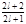at lower nodes on grid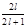at higher nodes on grid

Quantum solution for weak magnetic field is: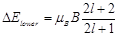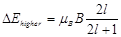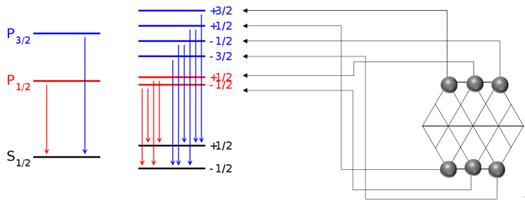Figure 3. Interference Grid and Zeeman Splitting.

When there is low external magnetic field, the interference grids are distorted. The Zeeman splitting is mainly based on the electron quantum location. Please note that mirror locations on the shell and opposite side of shell are equal while right hand nodes represent the side that reverses to the magnetic field.

3.5. Strong Magnetic Field Zeeman Splitting

The electrons are interacting with weak waves. Smallest unit of weak wave (1/137.036)* (1/137.036) is ½ spin. Methodologies of quantum can be used with help of the above weak wave to spin mapping. Since proton has three axes, the p cloud can either have 1/2 spin to interact with one axis, or 3/2 spin to interact with three axes.

4. Methods

4.1. Three Walls Water Interference

Materials:

a)  A digital camcorder

b)  A five to ten gallons water plastic bucket;

c)   Half gallon water.

Process:

Pour half gallon water into water bucket. Hold water bucket with left hand and put one corner of the bottom on a flat hard stable ground, tilt it 45 degrees.

Hold a digital camcorder with right hand and ready to take picture.

Shake the water bucket by knocking the bucket against the ground and take video for a few seconds while making knocking motions.

Select a picture with clear interference patterns.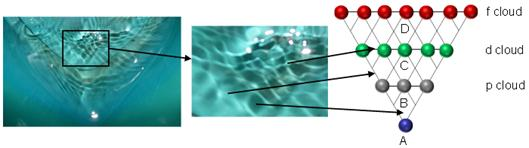Figure 4. Tri-wall Water Bucket Experiment.

The above picture provides valuable insights:

The interference pattern has a triangle shape and inner grids are diamond shaped when the waves are stabilized.

When mass distortions  collide with the axes of proton, the waves bounce back and form mass waves the same way as water bounce off the wall.

The electron interacts with axes of proton are similar to the water interactions with tri-wall water bucket. The left graph of FIG 4 represents a resonance point of electronic mass wave with proton tri-axes while each line of interference pattern in FIG 4 represents the resonance points of the waves. The intersections of three wave lines are quantum nodes (orbits) the electron has to follow. Once an electron associates with the node, it associates with the quantum numbers of the node.

4.2. Pauli Exclusion Principle

The Pauli exclusion principle with a single-valued many-particle wavefunction is equivalent to requiring the wavefunction to be antisymmetric. An antisymmetric two-particle state is represented as a sum of states in which one particle is in state |x> and the other in state |y>: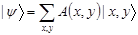The antisymmetry under exchange means that A(x,y) = −A(y,x). This implies that A(x,x) = 0, which is Pauli exclusion. It is true in any basis, since unitary changes of basis keep antisymmetric matrices antisymmetric, although strictly speaking, the quantity A(x,y) is not a matrix but an antisymmetric rank-two tensor.

Conversely, if the diagonal quantities A(x,x) are zero in every basis, then the wavefunction component: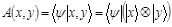is necessarily antisymmetric. To prove it, consider the matrix element: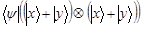This is zero, because the two particles have zero probability to both be in the superposition state. But this is equal to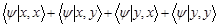The first and last terms on the right hand side are diagonal elements and are zero, and the whole sum is equal to zero. So the wavefunction matrix elements obey: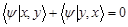Or

A(x, y) = -A(y, x)

5. Conclusions

Since the electrons are bouncing on octahedron shape protons, a heavy atom needs eight out most s/p cloud electrons to be stable. A proton has three internal structural axes. The mass wave of electron interacts with axes and form triangle grids. Each quantum state of electron is a node on the triangle grids. Weak interaction of electron and proton axes can explain Zeeman Splitting.

References

1. Cao, Zhiliang, and Henry Gu Cao. "Unified Field Theory and the Configuration of Particles." International Journal of Physics 1.6 (2013): 151-161.
2. Cao, Zhiliang, and Henry Gu Cao. "Unified Field Theory and Topology of Nuclei." International Journal of Physics 2, no. 1 (2014): 15-22.
3. Zhiliang Cao, Henry Gu Cao. Unified Field Theory. American Journal of Modern Physics. Vol. 2, No. 6, 2013, pp. 292-298. doi: 10.11648/j.ajmp.20130206.14.
4. Cao, Zhiliang, and Henry Gu Cao. "Unified Field Theory and the Hierarchical Universe." International Journal of Physics 1.6 (2013): 162-170.
5. Cao, Zhiliang, and Henry Gu Cao. "Non-Scattering Photon Electron Interaction." Physics and Materials Chemistry 1, no. 2 (2013): 9-12.
6. Cao, Zhiliang, and Henry Gu Cao. "SR Equations without Constant One-Way Speed of Light." International Journal of Physics 1.5 (2013): 106-109.
7. Cao, Henry Gu, and Zhiliang Cao. "Drifting Clock and Lunar Cycle." International Journal of Physics 1.5 (2013): 121-127.
8. Cao, Zhiliang, and Henry Gu Cao. "Unified Field Theory and Foundation of Physics." International Journal of Physics 2, no. 5 (2014): 158-164.
9. Mehul Malik, Mohammad Mirhosseini, Martin P. J. Lavery, Jonathan Leach, Miles J. Padgett & + et al. Direct measurement of a 27-dimensional orbital-angular-momentum state vector. Nature Communications, 2014, 5, doi:10.1038/ncomms4115
10. H. T. Yuan, M. B. Saeed, K. Morimoto, H. Shimotani, K. Nomura, R. Arita, Ch. Kloc, N. Nagaosa, Y. Tokura, and Y. Iwasa. Zeeman-Type Spin Splitting Controlled with an External Electric Field. Nat. Phys. 2013, 9, 563–569.
11. A. Rahimi-Iman, C. Schneider, J. Fischer, S. Holzinger, M. Amthor, S. Höfling, S. Reitzenstein, L. Worschech, M. Kamp, and A. Forchel. "Zeeman splitting and diamagnetic shift of spatially confined quantum-well exciton polaritons in an external magnetic field." Phys. Rev. B 84, 165325 – 2011, October
12. D. Kekez, A. Ljubiic & B. A. Logan. An upper limit to violations of the Pauli exclusion principle. Nature 348, 224-224 doi:10.1038/348224a0 (1990)
13. Zoran Hadzibabic. Quantum gases: The cold reality of exclusion. Nature Physics 6, 643-644 doi:10.1038/nphys1770 (2010)
14. June Kinoshita. Roll Over, Wolfgang? Scientific American 258, 25-28 doi:10.1038/scientificamerican0688-25 (1988)
15. Tony Sudbery. Exclusion principle still intact. Nature 348, 193-194 doi:10.1038/348193a0 (1990)
16. R. C. Liu, B. Odom, Y. Yamamoto & S. Tarucha. Quantum interference in electron collision. Nature 391, 263-265 doi:10.1038/34611 (1998)
17. George Gamow. The Exclusion Principle. Scientific American 201, 74-86 doi:10.1038/scientificamerican0759-74 (1959)
18. B. Poirier, Chem. Phys. 370, 4 (2010).
19. A. Bouda, Int. J. Mod. Phys. A 18, 3347 (2003).
20. P. Holland, Ann. Phys. 315, 505 (2005).
21. P. Holland, Proc. R. Soc. London, Ser. A 461, 3659 (2005).
22. G. Parlant, Y.-C. Ou, K. Park, and B. Poirier, "Classical-like trajectory simulations for accurate computation of quantum reactive scattering probabilities," Comput. Theor. Chem. (in press).
23. D. Babyuk and R. E. Wyatt, J. Chem. Phys. 124, 214109 (2006).
24. Jeremy Schiff and Bill Poirier. Quantum mechanics without wavefunctions. THE JOURNAL OF CHEMICAL PHYSICS 136, 031102 (2012)
25. J. von Neumann, Mathematical Foundations of Quantum Mechanics (Princeton University Press, Princeton, NJ, 1932).
26. D. Bohm, Phys. Rev. 85, 166 (1952).
27. P. R. Holland, The Quantum Theory of Motion (Cambridge University Press, Cambridge, England, 1993).
28. R. E. Wyatt, Quantum Dynamics with Trajectories: Introduction to Quantum Hydrodynamics (Springer, New York, 2005).
29. H. Everett III, Rev. Mod. Phys. 29, 454 (1957).
30. M. F. González, X. Giménez, J. González, and J. M. Bofill, J. Math. Chem. 43, 350 (2008).
31. Oganessian, Yu. T. et al. (2002). Results from the first 249Cf+48Ca experiment. JINR Communication (JINR, Dubna). http://www.jinr.ru/publish/Preprints/2002/287(D7-2002-287)e.pdf.
32. Nash, Clinton S. (2005). "Atomic and Molecular Properties of Elements 112, 114, and 118". Journal of Physical Chemistry A 109 (15): 3493–3500.
33. K.Umemoto, S.Saito, Electronic configurations of superheavy elements,
34. Journal of the physical society of Japan, vol.65, no.10, 1996, p.3175-3179
35. Hartmut M. Pilkuhn, Relativistic Quantum Mechanics, Springer Verlag, 2003.
36. E.Loza, V.Vaschenko. Madelung rule violation statistics and superheavy elements electron shell prediction. http://arxiv-web3.library.cornell.edu/abs/1206.4488

 Contents 1. 2. 2.1. 2.2. 2.3. 2.4. 2.5. 2.6. 2.7. 2.8. 2.9. 2.10. 3. 3.1. 3.2. 3.3. 3.4. 3.5. 4. 4.1. 4.2. 5.
Article ToolsAbstractPDF(299K)